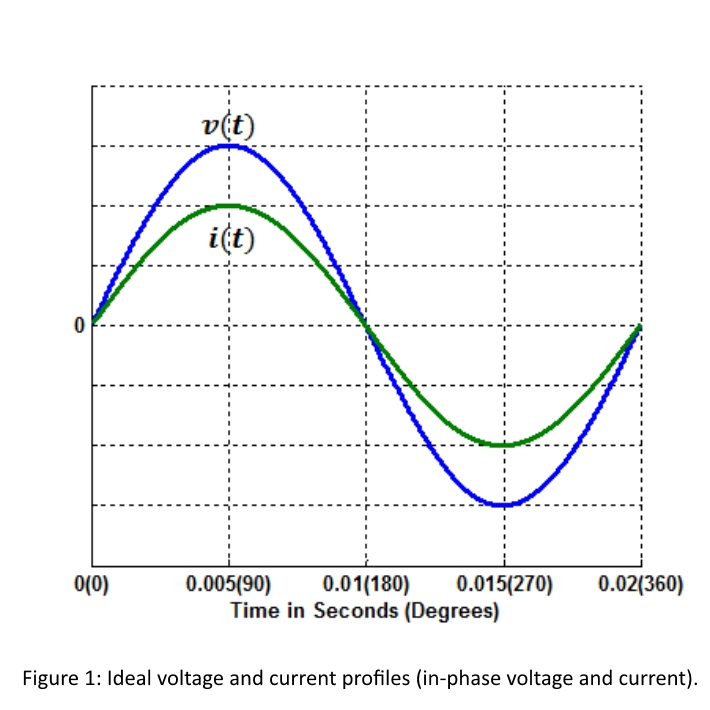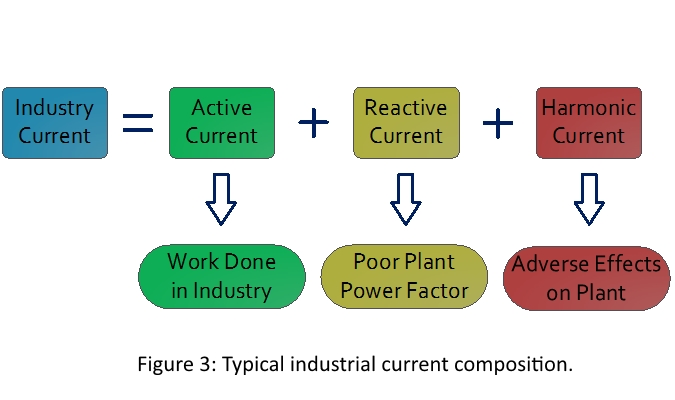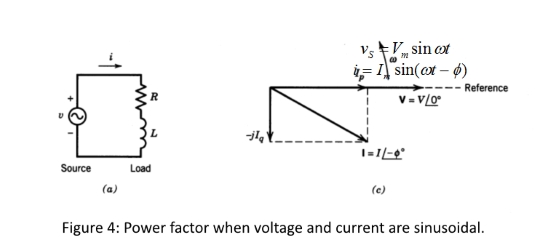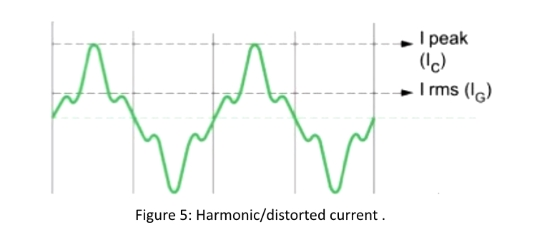# Knowledge Center

## Power Quality at Glance

#### Power Quality:

Power quality is related to the quality of power being supplied or consumed. It is maintained when:

• The State Electricity Board (EB) or Utility provides sinusoidal voltage at rated magnitude and rated frequency to its consumers
• Consumers draw sinusoidal current that is in-phase with the input supply voltage.

EB can assure power quality from their side only when its consumers do meet certain power quality standards (such as, IEEE 519-1992 and/or IEEE 519-2014). Recently, several EBs across the globe have started imposing strict regulations on quality of current, a customer can draw from the supply lines to maintain a healthy power distribution system.

Ideally, the input supply voltage and load current should have the following characteristics

• Voltage: rated magnitude with sinusoidal wave shape and rated frequency
• Current: sinusoidal wave shape at fundamental frequency and in-phase with the voltage

The above requirements can be illustrated as shown in the graph:

#### Major Power Quality Issues Related to Load Currents:

• Unbalanced Load Currents and Excessive Neutral Current
• Low Power Factor (LT Side and HT Side)
• HarmonicsThe balanced three-phase three-wire system is one having equal magnitude of currents in all the phases and having 120o phase angle difference between each of them. Unbalanced is characterized as currents (also applicable to the voltage) having unequal magnitude in one or more phase/line currents. The currents may also have different phase angle difference among one or more phases. In case of three-phase four-wire system (with neutral conductor), the unbalance results in neutral current flow. Higher the unbalance, higher would be the neutral current. Main reason for the unbalance current flow in a system is connection of different single-phase loads in one or more phases. Below figure illustrates unbalanced and neutral current profiles.

#### The unbalance can have following adverse effects on the system:

• Unbalance currents lead to unbalanced voltages
• Transformer over heating due to negative sequence currents/voltages
• Diesel Generators overheating and tripping due to unbalanced loading
• Excess neutral currents causing neutral voltage shifts and/or burns at the transformer
• Torsional vibrations and bearing failures in motors, and so.The power electronic devices (such as, variable frequency drives-VFDs, phase controlled rectifiers, choppers, and battery chargers) and other major electrical loads in the industries draw currents that have three main components:

• Active current at rated frequency,
• Reactive current at rated frequency, and
• Harmonic currents at higher frequencies (commonly at multiples of rated fundamental frequency).

Among these three parts, the active current is responsible for the actual work done in factory/plant, while the remaining two parts are just circulating between EB & load, and do not contribute to any useful work. These two non-active currents, however, have significant impact on the other connected loads and distribution system.

• Reactive current is responsible for the poor plant power factors and attracts penalty from the EB.
• Harmonic currents cause adverse effects on other loads in a plant and EB voltage quality..Power factor can be defined as a quantitative measure to estimate how effectively the active power is being utilized by the loads. Mathematically, it is expressed as:

Once the above parameters are known, the plant total harmonic current (IH) in amperes can be calculated as: $$Power Factor = PF = {{{Active Power} \over Apparent Power}} = {{{P} \over S}}$$

Where, P is active power and S is apparent power which is product of RMS voltage and net RMS current. When the load is pure resistive, both voltage and current will be in-phase (similar to Fig. 1). In such a case, there will not be any reactive current. That is, both P and S will be equal, and the power factor would be 1 (best possible scenario).When the voltage and current are sinusoidal, power factor is defined as cosine angle between voltage and current. Due to inductive loads (such as, motors), the current drawn from the system lags the voltage by angle φ The active power and power factor in this case are given as:

$$P = Vrms.Irms.\cos(φ)$$ $$Power Factor = {{{P} \over S}} = {{{Vrms.Irms.\cos(φ)} \over Vrms.Irms}} = {{\cos(φ)}}$$

Note that when there are excessive capacitive banks, the current may lead voltage by angle . This case also results in poor power factor.

When the voltage is pure sinusoidal and current is non-sinusoidal (distorted), power factor is defined by the below expression:

$$Power Factor = {{{I1,rms} \over Irms}} = \cos(θ1-φ1) = distributionfactor \times displacementpowerfactor$$

And, in the worst-case scenario, when both voltage and current are non-sinusoidal (distorted), the power factor is called as true power factor and is defined as:

$$TruePowerFactor = {{{\sum_P} \over Vrms.Irms}}$$

Where the total P is determined as summation of all powers resulted from individual harmonic currents.

#### Low Power Factor on LT Side

##### Challenges:
• Dynamic load changes
• Slow response of APFC panels in switching ON/OFF of capacitor banks
• Lack of step-less power factor correction mechanism
##### Effects:
• Loss of power factor incentives and/or subjected to penalties
• Excess energy bills in kVAH billing systems

#### Low Power Factor on HT Side

##### Challenges:
• Transformer has voltage and current dependent reactive power requirements.
• Difficult to meet varying transformer reactive power requirements at all times.
• Fixed capacitor banks cause excess leading KVARH during lite/no-load conditions.
• Lack of fixed capacitor banks cause lagging KVARH in spite of unity PF on LT side.
##### Effects:
• Loss of power factor incentives and/or subjected to penalties
• Excess energy bills in KVAH billing systems

Harmonics in the current or voltage are components at a multiple of fundamental frequency of the system. They are generated mainly due to turn on and off (switching) operation of power electronics-based system, for example, variable frequency drives-VFDs, phase-controlled rectifiers, choppers, battery chargers, televisions, printers, laptops, CFLs and so on. Fig. 5 shows a profile of distorted current that has harmonics. Comparing Fig. 1 and Fig. 5, one can visualize from the waveshapes that any waveform (current or voltage) that is not pure sinusoidal generally contains some harmonics. Note in Fig. 5, the peak current has larger magnitude compared the RMS current for the given waveform.

#### Key effects of higher level of harmonic currents could be:

• Increased heating of transformers, motors and cables
• Reduced plant efficiency
• Protective devices malfunctioning leading to increased down time
• Reduced life span of costly and sensitive plant equipment due to increased stresses
• Possible malfunction of other nearby sensitive equipment
• Poor efficiency, overheating, frequent failures, and reduced lifetime of the upstream utility equipment (such as, transmission lines, transformers, and substations)